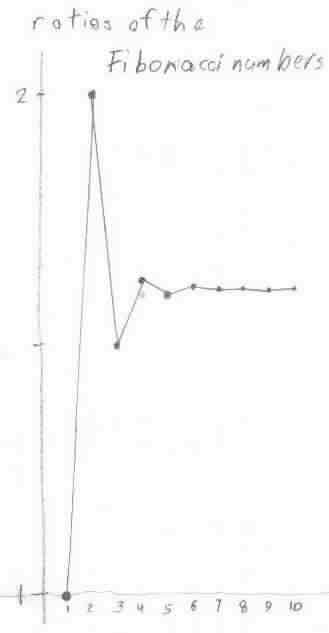Geoffrey works on the Fibonacci numbers

Geoffrey worked on the Fibonacci numbers and got their ratios. He graphed the ratios, and saw they formed a sequence that was alternating and approached some number 1.618033... He graphed the ratios:In Wells' "Dictionary of Curious and Interesting Numbers" he found that (Sqrt 5 +1)/2 = 1.61803... , was an irrational number, and was called The Divine Proportion or The Golden Mean.

Don and Geoffrey wrote a program in basic to get the ratios. Then that evening, Geoffrey and his Dad wrote a program in Mathematica to get the Fibonacci numbers. The following 9 lines formed the input, the output was the first 22 Fibonacci numbers below:
a=1;
b=1; Print[a];
Print[b];
Do[s=a+b;
Print[s];
a=b;
b=s,
{i,1,20}]

1, 1, 2, 3, 5, 8, 13, 21, 34, 55, 89, 144, 233, 377, 610, 987, 1597, 2584, 4181, 6765, 10946, 17711,

To Geoffrey's work on powers of powers
To Geoffrey's work on the area under curves and the integral
To Geoffrey's work on infinite series
To Geoffrey's work on graphs
To Geoffrey's work on the 6 trig functions
To other discoveries
To order Don's materials
Mathman home